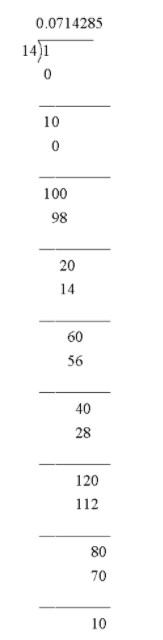QuestionAnswers

# Write the decimal expansion of $\dfrac{1}{{14}}$ up to $7$ decimal places.

Hint:The decimal expansion of a number is its representation in base $10$ (that is in the decimal system). In this system, each "decimal place" consists of a digit 0-9 arranged such that each digit is multiplied by a power of $10$, decreasing from left to right, and with a decimal place indicating the ${10^0} = {1^{st}}$ place.The digits after point are similarly expressed such that each digit is multiplied by a negative power of $10$.To find the decimal expansion of a given number we need to divide the numerator by denominator and get the quotient up to $7$ decimal place.

The given number is $\dfrac{1}{{14}}$. We need to find the decimal expansion of the number up to 7 decimal places.
We have to convert a fraction to a decimal is just a division operation. So the fraction $\dfrac{1}{{14}}$ means $1 \div 14$.
Then we get,Here we see that after carrying division $8$ times we return with a remainder $10$ which is the dividend at the second step of division continuing after 6 steps. Thus the string $714825$ respects in the quotient infinitely often. Thus the decimal expansion of ​$\dfrac{1}{{14}}$ looks like $\dfrac{1}{{14}} = 0.0714285714285....$(Here remainder is never $0$).
Since the number after in the ${8^{th}}$ decimal place is $7$(greater than $5$) by round of rule the ${7^{th}}$ place digit will be $5 + 1 = 6$.
Thus we can make it up to $7$ decimal places,$\dfrac{1}{{14}} = 0.0714286$
Now, we need to express this as decimal expansion so we get,
$0.0714286 = 0 \times {10^{ - 1}} + 7 \times {10^{ - 2}} + 1 \times {10^{ - 3}} + 4 \times {10^{ - 4}} + 2 \times {10^{ - 5}} + 8 \times {10^{ - 6}} + 6 \times {10^{ - 6}}$

Note:A decimal representation of a non-negative real number r is an expression in the form of a series, traditionally written as a sum
$r = \sum\limits_{i = 0}^\infty {\dfrac{{{a_i}}}{{{{10}^i}}}}$
where ${a_0}$ is a nonnegative integer, and ${a_1},{a_{2,}}........$ are integers satisfying $0 \leqslant {a_i} \leqslant 9$ , called the digits of the decimal representation.
Rules of rounding a number up to a certain decimal places:
If the number you are rounding is followed by $5,{\text{ }}6,{\text{ }}7,{\text{ }}8,{\text{ or }}9$ round the number up.
If the number you are rounding is followed by $0,{\text{ }}1,{\text{ }}2,{\text{ }}3,{\text{ or }}4$ leave the rounded number as it is.